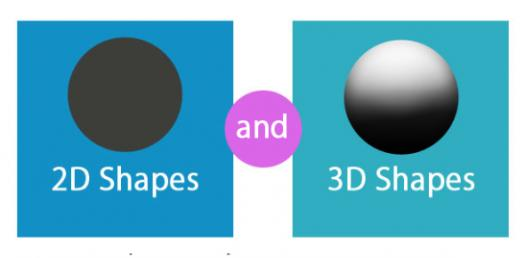# Test Your Knowledge About 2D And 3D Shapes! Trivia Quiz

6 Questions | Total Attempts: 66SettingsTest Your Knowledge About 2d And 3D Shapes! The main difference between these two shapes is the number of measurement where one has only the height and length whereas the other has the height, length and width. Do give it a try and get to see how well you know these shapes. All the best as you tackle it!

Questions and Answers
• 1.
Tessellation means ..........
• A.

That at least 1 shape must touch sides with another

• B.

That there is no gaps or no overlapping between shapes

• C.

That there must be gaps between shapes

• 2.
Right angled triangles can be tessellated to make patterns
• A.

True

• B.

False

• 3.
A cube has _____ faces.
• 4.
A tetrahedron has which of the following?
• A.

4 faces, 6 edges, 5 vertices

• B.

4 faces, 6 edges, 4 vertices

• C.

5 faces, 8 edges, 6 vertices

• D.

6 faces, 12 edges, 8 vertices

• 5.
An octahedron is a 2D shape and has equal sides and equal angles.
• A.

True

• B.

False

• 6.
A cuboid is a different name for a rectangle.
• A.

True

• B.

False

Related Topics